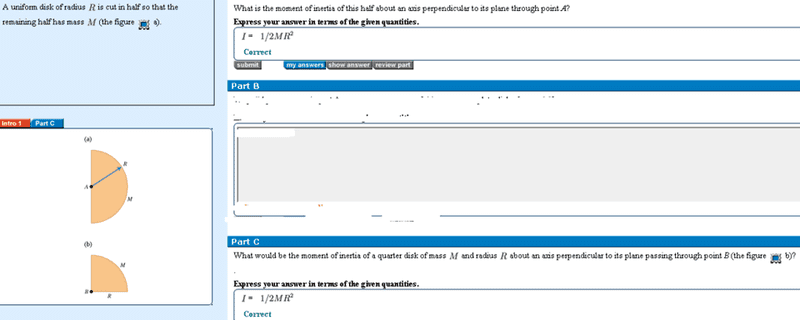# Moment of inertia?

## Homework Statement## Homework Equations

I=$$\Sigma$$mr^2

## The Attempt at a Solution

Our teacher said we don't need to memorize the Moment of inertia formulas because there is always a table we can look up for most objects. However we ran into problem in the HW.

Where does the 1/2 come from? Is that something we were just suppose to know? And why is I is the same for a whole circle or 1/2 a circle or even 1/4 of a circle?

alphysicist
Homework Helper
Hi kyin01,

These facts are straightforward to prove by performing the integral in the definition of the moment of inertia. However, since you say your teacher told you to just memorize the formulas, perhaps you are in a non-calculus based class?

However, once you have done the integral and know that the moment of inertia of a solid disk is [tex]\frac{1}{2}MR^2[/itex] for an axis perpendicular to the disk and through it's center, here is the reason the other two cases (semicircle and quadrant) have their formulas.

So imagine that you have an entire disk. Since it is made up of two identical halves (including their relationship to the axis), the moment of inertia of each half must be half the moment of inertia of the whole disk.

Similarly, the moment of inertia of each quadrant must equal one-fourth the moment of inertia of the entire disk.

Can you see how these will lead to (1/2)MR^2 for all three cases?

so if the moment of inertia must equal 1/4 the moment of inertia of the entire disk why isn't it 1/8MR^2?

alphysicist
Homework Helper
kyin01,

Because the mass of the quadrant is only one-fourth the mass of the whole disk.

If radius is R=1 and M=4 for the whole disk, I=(1/2)MR^2 = 2 kg m^2.

Broken up into four pieces, each piece has a mass of M=1, so

I=(1/2) M R^2=(1/2)(1)R^2 = 0.5 kg m/^2 which is one fourth the original.